## A-Sequence

N.B. A detailed on-line essay by S. Finch was the starting point for this entry.

An Infinite Sequence of Positive Integerssatisfying(1)

is an-sequence if nois the Sum of two or more distinct earlier terms (Guy 1994). Erdös (1962) proved(2)

Any-sequence satisfies the Chi Inequality (Levine and O'Sullivan 1977), which gives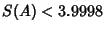. Abbott (1987) and Zhang (1992) have given a bound from below, so the best result to date is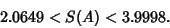(3)

Levine and O'Sullivan (1977) conjectured that the sum of Reciprocals of an-sequence satisfies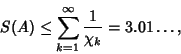(4)

where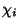are given by the Levine-O'Sullivan Greedy Algorithm.

References

Abbott, H. L. On Sum-Free Sequences.'' Acta Arith. 48, 93-96, 1987.

Erdös, P. Remarks on Number Theory III. Some Problems in Additive Number Theory.'' Mat. Lapok 13, 28-38, 1962.

Finch, S. Favorite Mathematical Constants.'' http://www.mathsoft.com/asolve/constant/erdos/erdos.html

Guy, R. K.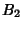-Sequences.'' §E28 in Unsolved Problems in Number Theory, 2nd ed. New York: Springer-Verlag, pp. 228-229, 1994.

Levine, E. and O'Sullivan, J. An Upper Estimate for the Reciprocal Sum of a Sum-Free Sequence.'' Acta Arith. 34, 9-24, 1977.

Zhang, Z. X. A Sum-Free Sequence with Larger Reciprocal Sum.'' Unpublished manuscript, 1992.